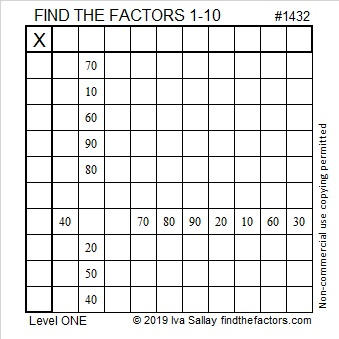# 1432 and Level 1

If you can count by tens, then you can solve this Level 1 puzzle. I dare you to prove me wrong!Print the puzzles or type the solution in this excel file:  10 Factors 1432-1442

Here is some information about the puzzle number, 1432:

• 1432 is a composite number.
• Prime factorization: 1432 = 2 × 2 × 2 × 179, which can be written 1432 = 2³ × 179.
• 1432 has at least one exponent greater than 1 in its prime factorization so √1432 can be simplified. Taking the factor pair from the factor pair table below with the largest square number factor, we get √1432 = (√4)(√358) = 2√358.
• The exponents in the prime factorization are 3 and 1. Adding one to each exponent and multiplying we get (3 + 1)(1 + 1) = 4 × 2 = 8. Therefore 1432 has exactly 8 factors.
• The factors of 1432 are outlined with their factor pair partners in the graphic below.1432 is 21212 in BASE 5 because
2(5⁴) + 1(5³) + 2(5²) + 1(5¹) + 2(5⁰) = 1432

This site uses Akismet to reduce spam. Learn how your comment data is processed.